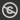Science, Maths & Technology
Free course

# Modelling and estimation## Course reviews

This free course is concerned with modelling and estimation and looks in particular at the binomial distribution. Section 1 starts by defining probability, introduces relevant notation and briefly discusses basic properties of probabilities. The section concludes by considering some of the general features of and ideas about modelling discrete random variables. Section 2 looks at one particular probability model for discrete data, the binomial distribution. Section 3 investigates how data can be used to estimate the probability of success in a single Bernoulli trial and introduces maximum likelihood estimation.

## Course learning outcomes

After studying this course, you should be able to:

• estimate a probability given data, and calculate a probability when assumptions about the symmetry of an object or situation can be made
• understand how probabilities of outcomes are encapsulated in the probability mass function (p.m.f.) of models for discrete data
• understand the meaning of the term Bernoulli trial, which describes a single statistical experiment for which there are two possible outcomes, often referred to as 'success' or 'failure'
• calculate binomial probabilities
• appreciate that the method of maximum likelihood estimation is an important way of estimating a parameter.

First Published: 19/12/2017

Updated: 18/04/2018

Skip Rate and Review